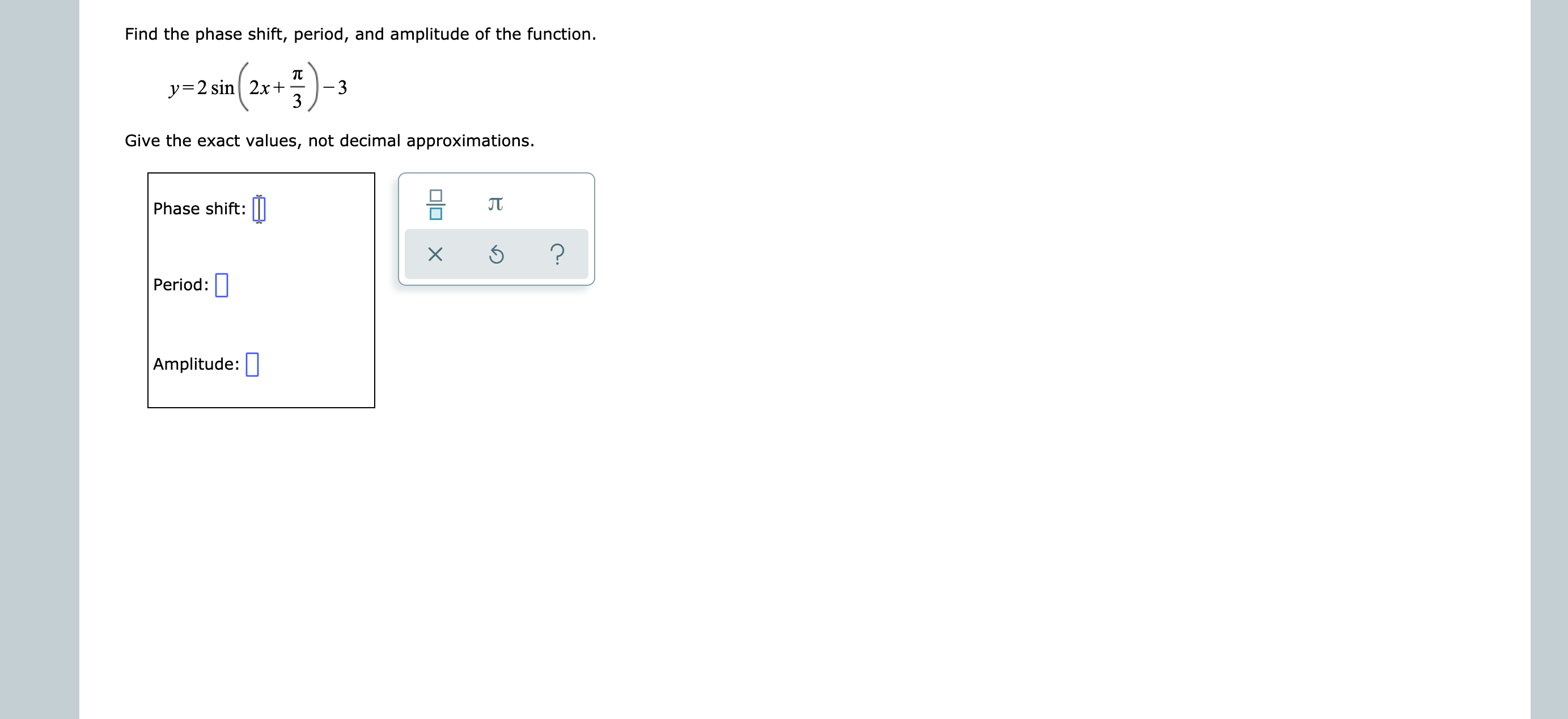# Find the phase shift, period, and amplitude of the function.y 2 sin 2x+33Give the exact values, not decimal approximations.JTPhase shift:?XPeriod:Amplitude:

Question
3 views

see attachmenthelp_outlineImage TranscriptioncloseFind the phase shift, period, and amplitude of the function. y 2 sin 2x+ 3 3 Give the exact values, not decimal approximations. JT Phase shift: ? X Period: Amplitude: fullscreen
check_circle

Step 1

Factor out 2.

Step 2

Phase shift = c

Comparing with standard form we get a= 2 , b=2 , c= -pi/6 and d=-3

Answer: Phase shift = c = -pi/6

Step 3

Period = 2pi/b

Period = 2pi/2= p...

### Want to see the full answer?

See Solution

#### Want to see this answer and more?

Solutions are written by subject experts who are available 24/7. Questions are typically answered within 1 hour.*

See Solution
*Response times may vary by subject and question.
Tagged in

### Other